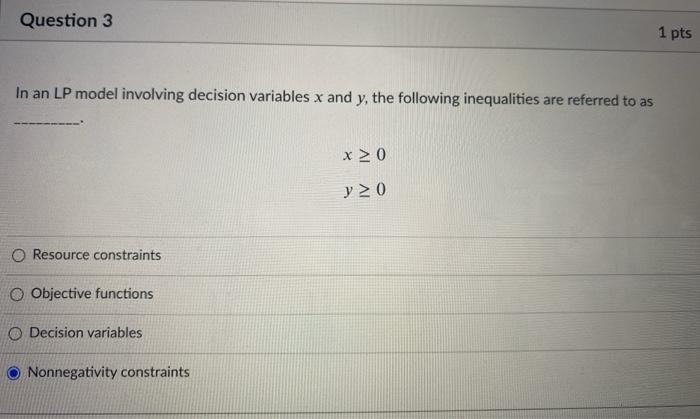# Question Question 3 1 pts In an LP model involving decision variables x and y, the following inequalities are referred to as x > 0 y > 0 Resource constraints O Objective functions O Decision variables Nonnegativity constraintsIERQWH The Asker · Other MathematicsTranscribed Image Text: Question 3 1 pts In an LP model involving decision variables x and y, the following inequalities are referred to as x > 0 y > 0 Resource constraints O Objective functions O Decision variables Nonnegativity constraints
More
Transcribed Image Text: Question 3 1 pts In an LP model involving decision variables x and y, the following inequalities are referred to as x > 0 y > 0 Resource constraints O Objective functions O Decision variables Nonnegativity constraints